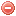# Definition

The vapor pressure deficit $$\Delta{e}$$ is the difference between saturation $$e_s$$ and actual vapor pressure $$e_a$$

# Formula

The vapor pressure deficit $$\Delta{e}$$ [kPa] can be calculated using temperature and relative humidity as follows (cf. Allen et al. 1998):

$\Delta{e}=e_s-e_a$

with

$e_s=0.6108\cdot e^{\frac{17.27\cdot{T}}{T+237.3}}$

and

$e_a=e_s\cdot{\dfrac{H}{100}}$

where $$T$$ is temperature [°C] and $$H$$ [%] relative humidity.

However, using mean air temperature as above results in a lower estimate of $$e_s$$, thus in a lower vapor pressure deficit. It would therefore be more appropriate to use, if available, maximal and minimum temperature for calculating $$e_s$$, as follows (Allen et al. 1998):

$e_s=\dfrac{1}{2}\bigg({0.6108\cdot e^{ \frac{17.27\cdot{T_{max}}}{T_{max}+237.3}}+0.6108\cdot e^{\frac{17.27\cdot{T_{min}}}{T_{min}+237.3}}}\bigg)$

where $$T_{max}$$ is maximal temperature [°C] and $$T_{min}$$ minimal temperature [°C].

NB: The conversion between kiloPascals and millimeters of mercury is as follows: 1 [kPa] = 7.500616827042 [mmHg]

# Reference

Allen et al. (1998)

### Bibliography SearchNo results!

No results were found. Are you sure you searched for a tag?

### Symbols

 Variable Description Unit $$T$$ air temperature °C $$T_{dew}$$ dew point temperature °C $$H$$ air humidity % $$P$$ rainfall mm $$U$$ windspeed m/s $$w$$ days since last rain (or rain above threshold) d $$rr$$ days with consecutive rain d $$\Delta t$$ time increment d $$\Delta{e}$$ vapor pressure deficit kPa $$e_s$$ saturation vapor pressure kPa $$e_a$$ actual vapor pressure kPa $$p_{atm}$$ atmospheric pressure kPa $$PET$$ potential evapotranspiration mm/d $$r$$ soil water reserve mm $$r_s$$ surface water reserve mm $$EMC$$ equilibrium moisture content % $$DF$$ drought factor - $$N$$ daylight hours hr $$D$$ weighted 24-hr average moisture condition hr $$\omega$$ sunset hour angle rad $$\delta$$ solar declination rad $$\varphi$$ latitude rad $$Cc$$ cloud cover Okta $$J$$ day of the year (1..365/366) - $$I$$ heat index - $$R_n$$ net radiation MJ⋅m-2⋅d-1 $$R_a$$ daily extraterrestrial radiation MJ⋅m-2⋅d-1 $$R_s$$ solar radiation MJ⋅m-2⋅d-1 $$R_{so}$$ clear-sky solar radiation MJ⋅m-2⋅d-1 $$R_{ns}$$ net shortwave radiation MJ⋅m-2⋅d-1 $$R_{nl}$$ net longwave radiation MJ⋅m-2⋅d-1 $$\lambda$$ latent heat of vaporization MJ/kg $$z$$ elevation m a.s.l. $$d_r$$ inverse relative distance Earth-Sun - $$\alpha$$ albedo or canopy reflection coefficient - $$\Delta$$ slope of the saturation vapor pressure curve kPa/°C $$Cc$$ cloud cover eights $$ROS$$ rate of spread m/h $$RSF$$ rate of spread factor - $$WF$$ wind factor - $$WRF$$ water reserve factor - $$FH$$ false relative humidity - $$FAF$$ fuel availability factor - $$PC$$ phenological coefficient -

 Suffix Description $$-$$ mean / daily value $$_{max}$$ maximum value $$_{min}$$ minimum value $$_{12}$$ value at 12:00 $$_{13}$$ value at 13:00 $$_{15}$$ value at 15:00 $$_{m}$$ montly value $$_{y}$$ yearly value $$_{f/a}$$ value at fuel-atmosphere interface $$_{dur}$$ duration $$_{soil}$$ value at soil level

 Constant Description $$e$$ Euler's number $$\gamma$$ psychrometric constant $$G_{SC}$$ solar constant $$\sigma$$ Stefan-Bolzmann constant